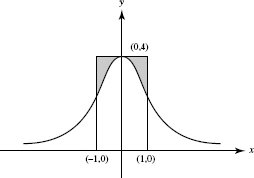# AP Calculus AB Practice Test 32

### Test Information9 questions18 minutes

Calculator Disallowed

1. The graph below shows the velocity of an object moving along a line, for 0 ≤ t ≤ 9.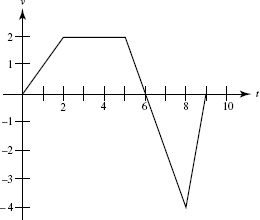The object is farthest from the starting point at t =

2. The graph below shows the velocity of an object moving along a line, for 0 ≤ t ≤ 9.At t = 8, the object was at position x = 10. At t = 5, the object's position was x =

3.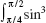α cos α dα is equal to

4.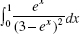equals

5. A differentiable function has the values shown in this table:

 x 2 2.2 2.4 2.6 2.8 3 f (x) 1.39 1.73 2.1 2.48 2.88 3.3

Estimate f (2.1).

6. If A =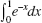is approximated using Riemann sums and the same number of subdivisions, and if L, R, and T denote, respectively left, right, and trapezoid sums, then it follows that

7. The number of vertical tangents to the graph of y2 = x - x3 is

8.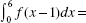9. The equation of the curve shown below is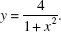What does the area of the shaded region equal?